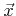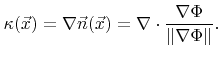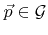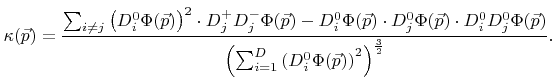Next: 3.4 Acceleration Techniques Up: 3.3 Approximations to Geometric Previous: 3.3.1 Surface Normal

## 3.3.2 Curvature

Solving the LS equation with a velocity field that is proportional to the curvature can be used for smoothing as will be demonstrated in Section 4.5. For an arbitrary pointthe (mean) curvature of the corresponding LS is defined as(3.24)

At grid pointsthe mean curvature can be approximated by(3.25)Next: 3.4 Acceleration Techniques Up: 3.3 Approximations to Geometric Previous: 3.3.1 Surface Normal

Otmar Ertl: Numerical Methods for Topography Simulation